# Model Lookup w/ Multiple Aggregate Models

I am trying to combine multiple aggregate models(task object) into one table via model lookup formula fields. The end goal is to have the main aggregate model display row data by user and have individual columns be the source of the other aggregate models (Image below).
• User entries are the main Task aggregate model.
• Each Type column is a separate Task aggregate model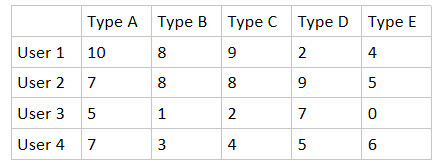The Model Lookup formula I am using on Type A is as follows:
VALUE(MODEL_LOOKUP("TypeA","countId","createdByName",{{CreatedBy.Name}}))

If I modify the formula to:
VALUE(MODEL_LOOKUP("TypeA","countId")) then I will see the first-row entry for TypeA displayed across all rows in my main task model. I've tried every combination for parameters 3 and 4, but nothing I do seems to make the models match up by the grouping field.

Column Task Model (Type A in this example)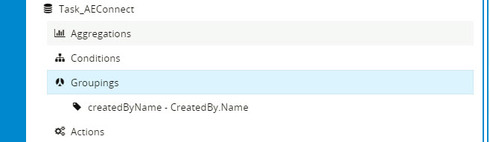Main Task Model (User in this example)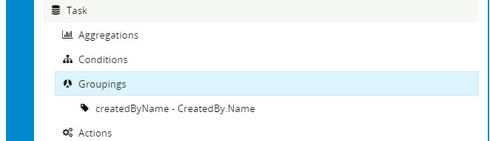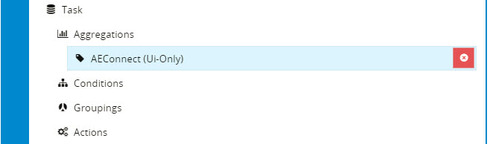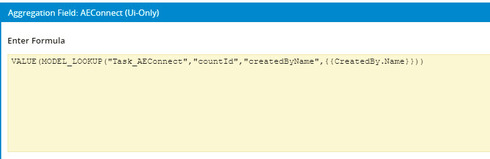• Can you use a basic model for the main model and have it display lookups to the agg models... I remember running into some issues doing model lookups FROM aggregate models, but it slips my mind what the issue was.
• That's getting closer, but the table is repeating values.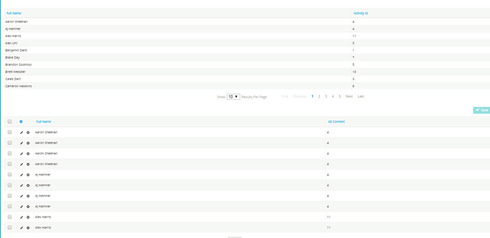• oh snap! I accidentally got it to work using an aggregate model as my main display.

The 4th parameter of the lookup statement needed the alias name from the main model, not the field API name. Woot!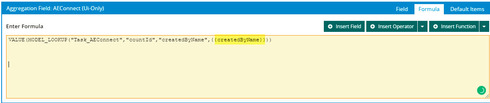• Nice!
• edited October 25, 2017
Cool!
• edited October 25, 2017
Cool!